# Fluid Dynamics¶

Important: Please read the installation page for details about how to install the toolboxes. $\newcommand{\dotp}{\langle #1, #2 \rangle}$ $\newcommand{\enscond}{\lbrace #1, #2 \rbrace}$ $\newcommand{\pd}{ \frac{ \partial #1}{\partial #2} }$ $\newcommand{\umin}{\underset{#1}{\min}\;}$ $\newcommand{\umax}{\underset{#1}{\max}\;}$ $\newcommand{\umin}{\underset{#1}{\min}\;}$ $\newcommand{\uargmin}{\underset{#1}{argmin}\;}$ $\newcommand{\norm}{\|#1\|}$ $\newcommand{\abs}{\left|#1\right|}$ $\newcommand{\choice}{ \left\{ \begin{array}{l} #1 \end{array} \right. }$ $\newcommand{\pa}{\left(#1\right)}$ $\newcommand{\diag}{{diag}\left( #1 \right)}$ $\newcommand{\qandq}{\quad\text{and}\quad}$ $\newcommand{\qwhereq}{\quad\text{where}\quad}$ $\newcommand{\qifq}{ \quad \text{if} \quad }$ $\newcommand{\qarrq}{ \quad \Longrightarrow \quad }$ $\newcommand{\ZZ}{\mathbb{Z}}$ $\newcommand{\CC}{\mathbb{C}}$ $\newcommand{\RR}{\mathbb{R}}$ $\newcommand{\EE}{\mathbb{E}}$ $\newcommand{\Zz}{\mathcal{Z}}$ $\newcommand{\Ww}{\mathcal{W}}$ $\newcommand{\Vv}{\mathcal{V}}$ $\newcommand{\Nn}{\mathcal{N}}$ $\newcommand{\NN}{\mathcal{N}}$ $\newcommand{\Hh}{\mathcal{H}}$ $\newcommand{\Bb}{\mathcal{B}}$ $\newcommand{\Ee}{\mathcal{E}}$ $\newcommand{\Cc}{\mathcal{C}}$ $\newcommand{\Gg}{\mathcal{G}}$ $\newcommand{\Ss}{\mathcal{S}}$ $\newcommand{\Pp}{\mathcal{P}}$ $\newcommand{\Ff}{\mathcal{F}}$ $\newcommand{\Xx}{\mathcal{X}}$ $\newcommand{\Mm}{\mathcal{M}}$ $\newcommand{\Ii}{\mathcal{I}}$ $\newcommand{\Dd}{\mathcal{D}}$ $\newcommand{\Ll}{\mathcal{L}}$ $\newcommand{\Tt}{\mathcal{T}}$ $\newcommand{\si}{\sigma}$ $\newcommand{\al}{\alpha}$ $\newcommand{\la}{\lambda}$ $\newcommand{\ga}{\gamma}$ $\newcommand{\Ga}{\Gamma}$ $\newcommand{\La}{\Lambda}$ $\newcommand{\si}{\sigma}$ $\newcommand{\Si}{\Sigma}$ $\newcommand{\be}{\beta}$ $\newcommand{\de}{\delta}$ $\newcommand{\De}{\Delta}$ $\newcommand{\phi}{\varphi}$ $\newcommand{\th}{\theta}$ $\newcommand{\om}{\omega}$ $\newcommand{\Om}{\Omega}$

This numerical tour explores fluid dynamics for image generation.

In :
from __future__ import division

import numpy as np
import scipy as scp
import pylab as pyl
import matplotlib.pyplot as plt

from nt_toolbox.general import *
from nt_toolbox.signal import *

import warnings
warnings.filterwarnings('ignore')

%matplotlib inline


## Velocity Flow Field¶

A velocity flow is simply a 2-D vector field $V = (V_i)_{i=1}^N \in \RR^{n \times n \times 2}$ where $V_i \in \RR^2$ is one of the $N=n \times n$ vectors at a position indexed by $i$.

It can be generated as a realization of Gaussian process. The blurring creates correlations in the flow.

In :
n = 128 # grid size

In :
t = np.linspace(0,1,n)
[Y,X] = np.meshgrid(t,t)
gamma = .05 # blurring size
xi1 = np.exp(-(X-Y)**2/gamma)
xi = lambda x: np.dot(np.dot(xi1,x),xi1)

In :
from numpy import random
n = 128
V = np.random.randn(n, n, 2)
for k in range(2):
V[:,:,k] = xi(V[:,:,k])


Subsampling display operator.

In :
from nt_toolbox.plot_vf import *
myplot = lambda V: plot_vf(V[np.ix_(np.arange(0,n,6),np.arange(0,n,6),np.arange(2))])


We can display the vector field using arrow.

In :
plt.figure(figsize = (7,7))
myplot(V)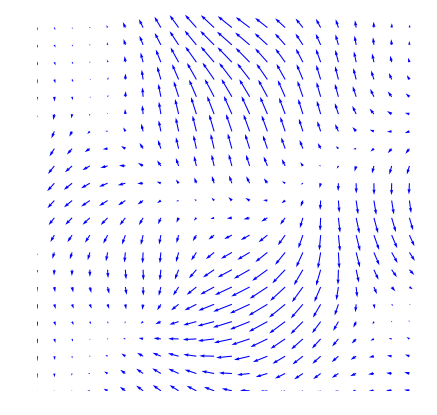We can renormalize the flow, which enhances the singularities. It defines $\tilde V$ as $\tilde V_i = V_i/\norm{V_i}$.

In :
normalize = lambda V: V/(np.repeat(np.maximum(1e-9*np.ones(np.shape(V)[:2]), np.sqrt(np.sum(V**2, 2)))[:,:,np.newaxis],2,axis=2))


Display.

In :
plt.figure(figsize = (7,7))
myplot(normalize(V))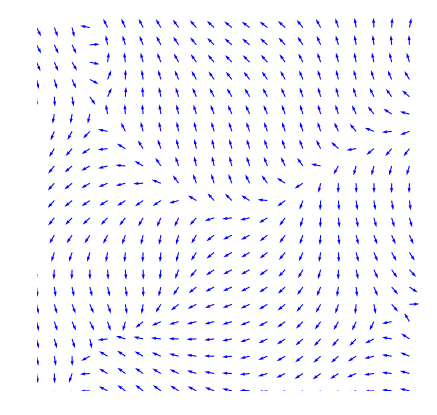## Incompressible Flows¶

An incompressible flow has a vanishing divergence. The set of vector incompressible flow defines a sub-space of $\RR^{n \times n \times 2}$ $$\Ii = \enscond{V}{ \text{div}(V)=0 } \qwhereq \text{div}(V) = \pd{V}{x_1} + \pd{V}{x_2} \in \RR^{n \times n}.$$ Here $\pd{}{x_s}$ for $s=1,2$ are finite differences approximation of the horizontal and vertical derivative operators (we suppose here periodic boundary conditions).

The orthogonal projection $U = \text{Proj}_{\Ii}(V)$ on $\Ii$ is computed by solving a Poisson equation $$U = V-\nabla A \qwhereq \Delta A = \text{div}(V).$$

This is especially simple for periodic boundary conditions since $A$ can be computed over the Fourier domain as $$\forall \om \neq 0, \quad \hat A(\om) = \frac{\hat Y(\om)}{\mu(\om)} \qwhereq Y = \text{div}(V) \qandq \mu(\om_1,\om_2) = -4 \sin(\om_1 \pi / n)^2 -4 \sin(\om_2 \pi / n)^2$$ and $\hat A(0)=0$.

Compute the kernel $\mu(\om)$.

In :
Y, X = np.meshgrid(np.arange(0,n),np.arange(0,n))
mu = np.sin(X*np.pi/n)**2
mu = -4*(mu + np.transpose(mu))
mu[0,0] = 1

In :
a = np.hstack((np.arange(1,n),))
b = np.hstack(([n-1],np.arange(n-1)))
Grad = lambda f: np.dstack( (f[a,:]-f, f[:,a]-f) )
Div = lambda v: v[b,:,0]-v[:,:,0] + v[:,b,1]-v[:,:,1]
Delta = lambda g: (g[a,:] + g[b,:] + g[:,a] + g[:,b]) - 4*g
DeltaV = lambda V: np.dstack( (Delta(V[:,:,0]), Delta(V[:,:,1]))  )


Computation of $A$.

In :
#from nt_toolbox.div import *
#A = lambda V: np.real(pyl.ifft2(pyl.fft2(Div(V[:,:,0], V[:,:,1], bound="per"))/mu))
A = lambda V: np.real(pyl.ifft2(pyl.fft2(Div(V))/mu))


Projection on incompressible flows.

In :
ProjI = lambda V: V + Grad(A(V))


Display $U=\text{Proj}_{\Ii}(V)$.

In :
U = ProjI(V)
plt.figure(figsize=(7,7))
myplot(U)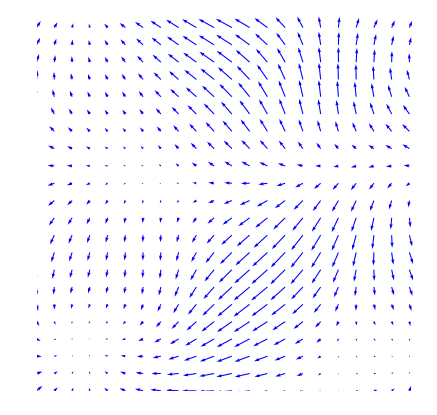Display $W=U-V$ the irrotational component of $V$.

In :
plt.figure(figsize=(7,7))
myplot(V-U)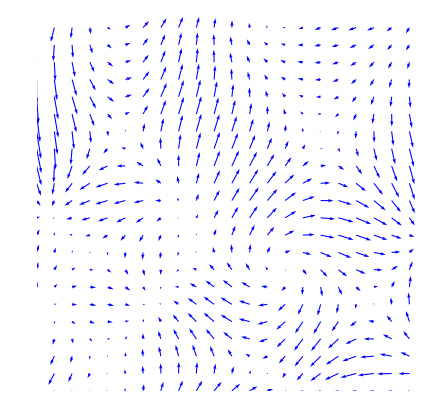Note that the decomposition $V=U+W$ is called the Hoge decomposition of the vector field.

## Image Advection Along the Flow¶

A flow defines a warping operator that transport the content of an image along the streaming of the flow.

We load an image $f$.

In :
f = load_image("nt_toolbox/data/hibiscus.bmp", 2*n)
f = f[np.ix_(np.arange(n-n//2,n+n//2),np.arange(n-n//2,n+n//2))]


Given some vector field $U$, the warping operator $f_1 = \Ww_U(f)$ along the flow is defined $$f_1(x) = f(x+U(x))$$ i.e. it advects the values of $f$ by the vector field $U$ to obtain the values of $f_1$.

We define $U$ as a scaled normalized incompressible flow.

In :
U = normalize( ProjI(V) )


Helper function: enforce periodicity.

In :
periodic = lambda P: np.concatenate(((P[:,:,0]%n)[:,:,np.newaxis], (P[:,:,1]%n)[:,:,np.newaxis]), axis=2)


Helper function: extend an image by 1 pixel to avoid boundary problems.

In :
extend1 = lambda f: np.hstack((f,f[:,]))
extend = lambda f: np.transpose(extend1(np.transpose(extend1(f))))


Helper function: bilinear interpolation on a grid.

In :
from scipy import interpolate

def myinterp(P1,f1,Pi):
return interpolate.griddata(P1, np.ravel(f1),(Pi[:,:,1],Pi[:,:,0]))


First we compute the initial and wraped grids.

In :
Y, X = np.meshgrid(np.arange(0,n),np.arange(0,n))
P = np.concatenate((X[:,:,np.newaxis],Y[:,:,np.newaxis]),axis=2)
Y1, X1 = np.meshgrid(np.arange(0,n+1),np.arange(0,n+1))
P1 = np.concatenate((np.ravel(Y1)[:,np.newaxis],np.ravel(X1)[:,np.newaxis]),axis=1)


Defines the warping operator $\Ww_U$.

In :
W = lambda f, U: myinterp(P1, extend(f), periodic(P - U))


Display a warped image $\Ww_{\rho U}(f)$ for some scaling $\rho$.

In :
rho = 2
plt.figure(figsize = (5,5))
imageplot(W(f, rho*U))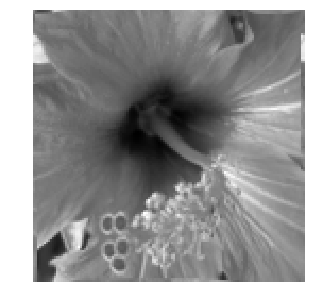Exercise 1

Display $\Ww_{\rho U}(f)$ for various values of $\rho$.

In :
run -i nt_solutions/graphics_5_fluids/exo1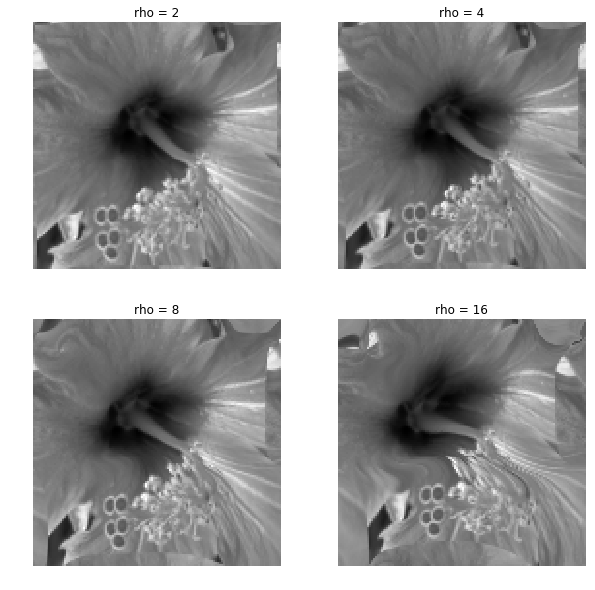In :
## Insert your code here.


Exercise 2

Define an iterative scheme via: $$f^{(\ell+1)} = \Ww_{\rho U}(f^{(\ell)}).$$ Display the result $f^{(\ell)}$, which corresponds approximately to solving an advection equation at time $t=\ell \rho$.

In :
run -i nt_solutions/graphics_5_fluids/exo2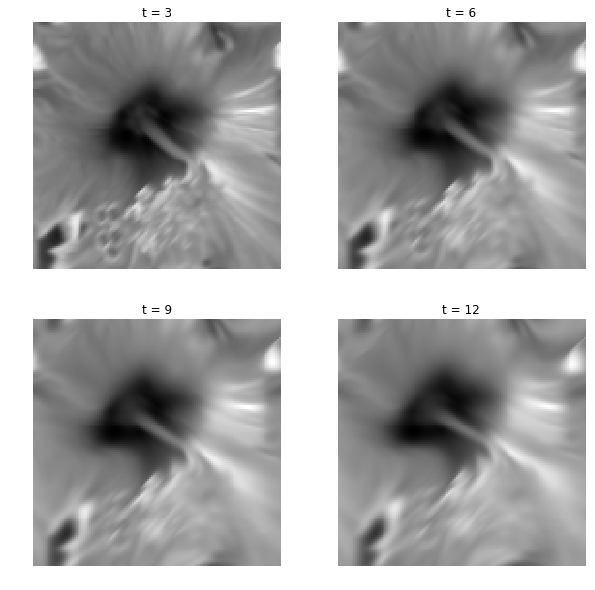In :
## Insert your code here.


## Fluid Dynamics¶

Fluid dynamics solves the incompressible Navier-Stokes equations to evolve in time the vector field.

We discribe here a simple algorithm introduced in:

J. Stam, Stable Fluids, SIGGRAPH'99, 1999, p. 121-128.

It proposes a semi-implicit scheme for the resolution of the Navier Stockes equations for the movement of incompressible fluids $$\pd{V}{t} = \text{Proj}_{\Ii}\pa{ -(V \cdot \nabla) V + \nu \Delta V + W }.$$ Here $\nu \geq 0$ is the viscosity of the fluid, $W$ is a source term, $\Delta$ is the Laplacian, and $-(V \cdot \nabla) V$ is the non-linear self-advection, where we have used the short-hand notation $V \cdot \nabla$ for the derivative operator along a flow $V$: $$(V \cdot \nabla)U = ( V_1 \pd{U_1}{x_1} + V_2 \pd{U_1}{x_2}, V_1 \pd{U_2}{x_1} + V_2 \pd{U_2}{x_2} ).$$

In order to visualize the flow, we also advect and diffuse along the flow a density $g$ of particules, which is a scalar field. Once $V$ has been computed, it follows a linear PDE $$\pd{g}{t} = -(V \cdot \nabla) g + \mu \Delta g + h$$ with some initial condition at time $t=0$, where $h$ is a source for the density.

In practice, we solve this PDE in parallel to the PDE for $V$.

In the following, we use $W=0$ and $h=0$ (no sources).

Set the viscosity $\nu$ for the velocity field.

In :
nu = 1/10
mu = nu


Extend the warping operator $\Ww_U$ to work with vector fields as input. This will apply $\Ww_U$ on each channel of the vector field (X and Y coordinates).

In :
#Wt = lambda V, U: np.concatenate((W(V[:,:,0],U)[:,:,np.newaxis],W(V[:,:,1],U)[:,:,np.newaxis]),axis=2)
Wt = lambda V, U: np.dstack( (W(V[:,:,0],U),W(V[:,:,1],U)) )


We discretize the PDE's using some time step $\tau$.

In :
tau = .03


The algorithm computes $V^{(\ell)}$ at iteration $\ell$ which is an approximation of the PDE solution at time $\ell \tau$. It is computed itertatively as $$\tilde V^{(\ell)} = \Ww_{\tau V^{(\ell)}}( V^{(\ell)} ) \qandq V^{(\ell+1)} = \text{Proj}_{\Ii}\pa{ \tilde V^{(\ell)} + \tau\nu\Delta \tilde V^{(\ell)} + \tau W }$$

It computes in parallel the evolution of the density as $$\tilde g^{(\ell)} = \Ww_{\tau V^{(\ell)}}( g^{(\ell)} ) \qandq g^{(\ell+1)} = \tilde g^{(\ell)} + \tau\nu\Delta \tilde g^{(\ell)} + \tau h$$

Set the initial field $V=V^{(0)}$ at time $t=0$.

In :
U = ProjI(V)


Set the initial density $g=g^{(0)}$ at time $t=0$.

In :
g = np.copy(f)


The first step is to advect the vector field $V$ and $g$ along the flow $V$ itself. This corresponds to an implict discretization of the term $-(V \cdot \nabla) V$.

In :
g = W (g, tau*U)
U = Wt(U, tau*U)


We implement the Laplacian using finite difference.

The second step is to diffuse the vector field and the density.

In :
U = U + tau*nu*DeltaV(U)
g = g + tau*mu*Delta(g)


The last step is to ensure incompressibility of $V$ by projecting on $\Ii$.

In :
U = ProjI(U)


Exercise 3

Compute the fluid dynamic by iterating these steps.

In :
run -i nt_solutions/graphics_5_fluids/exo3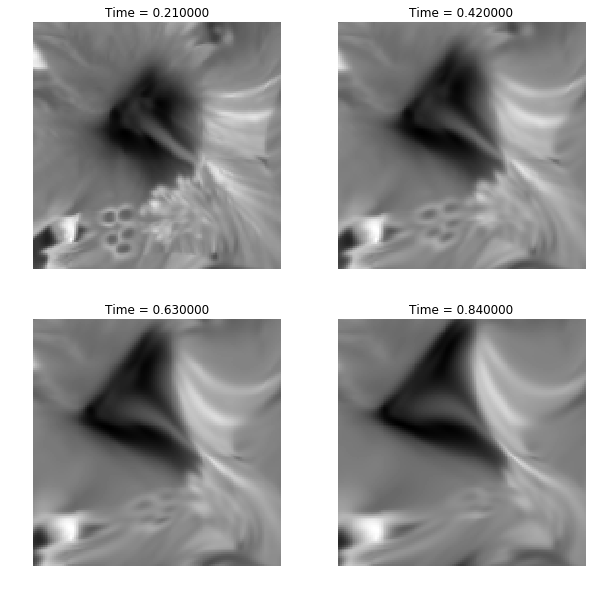In :
## Insert your code here.